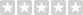Popular tags: Support, Resistance, Trend, Range, Fibonacci, Wave, Divergence, Multi time frames(MTF), Swing, Volume, Overbought, Oversold

Featured

# Reverse Engineering MACD for Tradingview

Availability: Intro Only, not for purchase
Price: Free
Qty:     - OR -(0/5)  0 reviews  |  Write a review

The Reverse Engineering MACD study is a trend following indicator based on analysis of MACD (Moving Average Convergence/Divergence) changes. This indicator consists of three main plots: PMACDeq, PMACDlevel, and PMACDsignal.

PMACDeq plot represents the price of the current bar at which its MACD value would be equal to the previous one. PMACDlevel plot represents the price of the current bar at which its MACD value would be equal to the specified one. PMACDsignal plot represents the price of the current bar at which its MACD value would be equal to its average.

These three plots are accompanied by an auxiliary plot showing averaged values of PMACDeq. Note that the type of average is the same as the specified for MACD calculation.

Input Parameters:

Price The price for which MACD is calculated.
Fast length The number of bars used to calculate the fast moving average.
slow length The number of bars used to calculate the slow moving average.
macd length The number of bars used to calculate the moving average of MACD.
macd level MACD value used in PMACDlevel calculation.

## Write a review

Your Review: Note: HTML is not translated!

Enter the code in the box below:Latest
Welcome to Patternsmart
Converting your indicator from one platform to another.